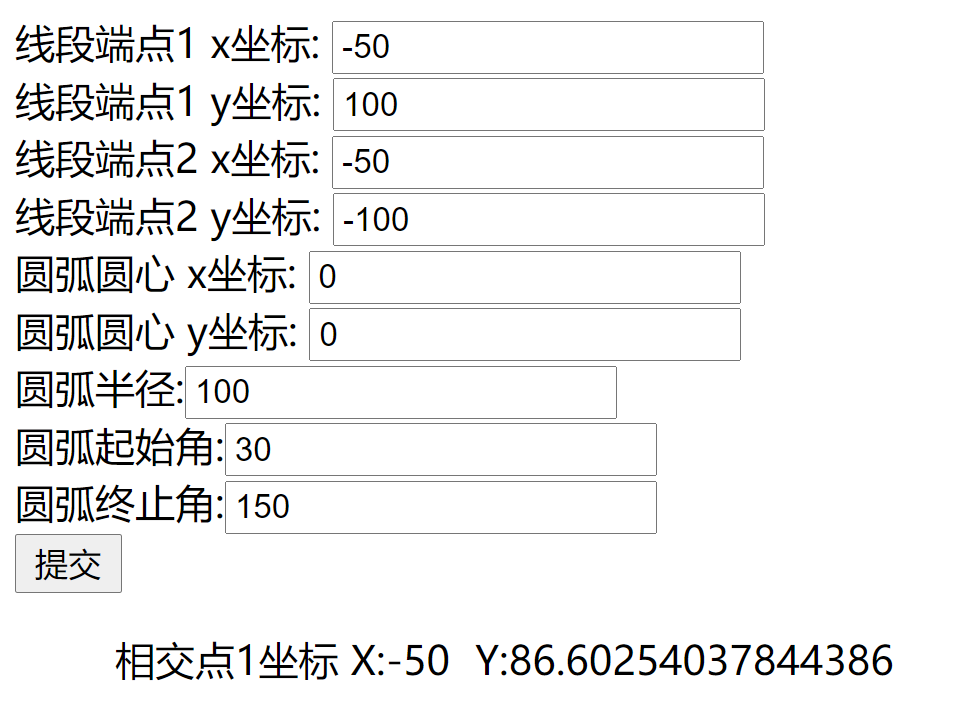# 求线段与圆弧的交点

最近面试遇到一个问题，就是已知一条线段的两个端点坐标A(x1,y1)，B(x2,y2)，和一个圆弧(起始点逆时针旋转到终止点的圆弧)的圆心坐标C(m,n)，半径r，起始角a1和终止角a2(起始角和终止角是指以x轴正方向为起点，逆时针转动的角度)，求线段和圆弧的交点。网上这方面的资料太少了，所以记录下来，希望给后人一些帮助。

解决这个问题，可以分为几步：

1.第一步求圆弧的圆心到直线（线段）的距离d  = |(Ax0 + By0 + C) / Math.sqrt((A^2 + B^2))|，A = Y2 - Y1，B = X1 - X2，C = X2*Y1 - X1*Y2，当d大于圆弧半径r时，说明线段和圆弧不可能相交，不用计算下面的了，直接返回；当d<=r时，进入第2步。

2.求出线段组成的直线和圆弧组成的圆的交点，结果可能1个或者2个交点。求直线和圆的交点就是求直线的方程y=kx+b和圆的方程(x-m)^2+(y-n)^2 = r^2组成的方程组的解。直线的方程可以通过带入线段两个坐标值求得，即y1=kx1+b和y2=kx2+b，带入得k=(y1-y2)/(x1-x2),b=(x1y2-x2y1)/(x1-x2)，这里要考虑斜率k存在和k不存在的情况(还有一种k=0可以当作k存在处理)。如果k存在，求得k,b之后再把直线方程代入圆的方程得(x-m)^2+(kx+b-n)^2=r^2，即(1+k^2)x^2+(2kb-2kn-2m)x+m^2+(b-n)^2-r^2=0这个二元一次方程的解，根据求根公式得x1 = (-b + sqrt(b^2 - 4ac)) / (2a), x2 = (-b - sqrt(b^2 - 4ac)) / (2a),其中a=1+k^2，b=2kb-2kn-2m，c=(b-n)^2-r^2，进而求出交点的坐标。如果k不存在则直线的方程等于x=x1，代入圆的方程得(x1-m)^2+(y-n)^2=r^2，即求y^2-2ny+n^2+(x1-m)^2-r^2=0，根据求根公式得y1 = (-b + sqrt(b^2 - 4ac)) / (2a), y2 = (-b - sqrt(b^2 - 4ac)) / (2a)，其中a=1，b=-2n，c=n^2+(x1-m)^2-r^2，进而求出交点坐标。

3.判断交点是否在线段上，判断点是否在线段上的方法可以通过判断点是否在以线段两个端点为对角线组成的矩形内(矩形的边分别垂直与x轴，y轴)，前提是点已经在线段组成的直线上。如果交点不在线段上，则返回空，否则进入下一步。

4.判断交点是否在圆弧上，我的方法是先根据圆弧的圆心，半径和起始角求出圆弧的起点坐标，公式为beginPoint.x = m+r*Math.cos(a1);beginPoint.y = n+r*Math.sin(a1);然后根据起始点和圆心坐标求出圆弧的起点角度startAngle(这里的起点角不是题目给出的起始角a1，这个起点角度的坐标系原点是圆心)，可以通过正切函数计算，这里的正切所得的角度都是小于90度的，我是再根据各个点坐标转换到以圆心为原点的坐标系后根据点的坐标在以圆心为原点的坐标系哪个象限确定角度的。同理求出终点角endAngle(startAngle可能大于andAngle，因为圆弧是从起点逆时针转到终点形成的)，然后再求出交点的角度angle。当startAngle<endAngle时，如果满足startAngle<=angle<=endAngle，则交点在圆弧上；当startAngle>endAngle时，如果满足angle>=startAngle || angle<=endAngle(因为angle分布在0~endAngle和startAngle~360度的区域范围)，则交点在圆弧上。

经过以上步骤即可求出线段和圆弧的交点，程序运行结果截图：https://download.csdn.net/download/wdglhack/13983942

12-3004-191万+
08-14560
08-111827
05-08586
04-098672
12-277023
08-21
05-132910
11-08596
10-17
01-16481
01-151万+
05-04126
10-11802
12-252414
©️2020 CSDN 皮肤主题: 大白 设计师:CSDN官方博客¥2 ¥4 ¥6 ¥10 ¥20余额支付 (余额：-- )扫码支付获取中扫码支付